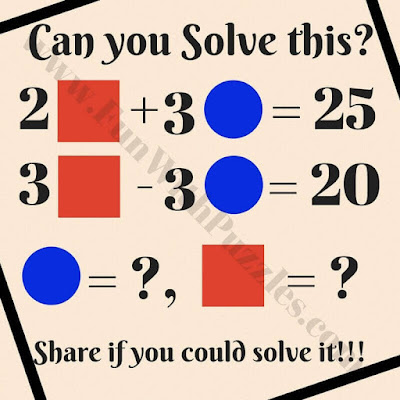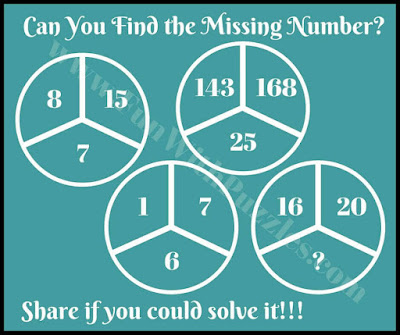## Saturday, August 31, 2019

There are endless possibilities with which Mathematical Logical puzzles can be created. Some of the puzzles are pure mathematical puzzles in which one has to calculate a given value according to math equations. However, there are many in which logic in mathematical equations is twisted and one has to first find this twisted logic and then has to solve the mathematical equation.
These Mathematical brain teasers with pictures which will require you to think first logical and then solve the given math question. In each of the below question to find the value of "?".
Links to the similar Maths Picture Brain Teasers are given after each Puzzle Picture Image. Do click on these links to solve similar Maths Picture Puzzles. Answers of these math riddles with explanation are given at the end. However please do post your answers in the comment section of this post. Also, let us know if your logic and explanation are different from our logical reasoning?1. What is the value of the missing number?2. Can you find the missing number?3. Can you find the value of Square and Rectangle?4. Can you solve these Maths Equations?5. Can you find the value of the missing number?

## Answers of Math Picture Brain Teasers

The solution of these Tricky Math Riddles is given as below.
A1) 27
Each number in the box is the multiplication of the outside numbers.

A2) 5
Required number=sum of the squares of the numbers on the left side - the sum of the squares of the numbers on the right side.

A3) Square = 9 , Circle =7/3
Explanation 2s + 3 c= 25............(1)
3s- 3c = 20....................(2)
on adding 1 & 2 , we have
3s= 45
s= 9 , put value of s in equation 1,we have c= 7/3.

A4) Value of cylinder= 3 , square=5, star = 2
Explanation:
2cy + sq= 11.....(1)
sq+st+cy = 10.............(2)
2 sq+st = 12................(3)
From equation 1 & 2 .
2cy = 11 - sq , Means cy = 11-sq /2
and st = 12-2 sq
putting values of cy and st in equation 3,
sq + 12 - 2sq + 11/2- sq/2 = 10
sq - 5/2 sq = 10 - 12 - 11/2,
-3/2 sq = -11/2 - 2 or
-3/2 sq =- 15/2,
sq= 5,
putting values we have cy= 3, st=2

A5) 4
Subtraction of the other two numbers in the circle.

1.1.